DraftKings Championship Odds
+250
2.5 to 1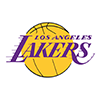Lakers
22.0% implied probability

+300
3 to 1Nets
19.3% implied probability

+600
6 to 1Clippers
11.0% implied probability

+600
6 to 1Bucks
11.0% implied probability

+1600
16 to 176ers
4.5% implied probability

+1800
18 to 1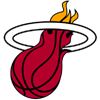Heat
4.1% implied probability

+1800
18 to 1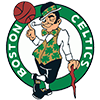Celtics
4.1% implied probability

+2000
20 to 1Nuggets
3.7% implied probability

+2200
22 to 1Mavericks
3.3% implied probability

+2500
25 to 1Jazz
3.0% implied probability

+4000
40 to 1Trail Blazers
1.9% implied probability

+4000
40 to 1Suns
1.9% implied probability

+4000
40 to 1Pacers
1.9% implied probability

+4500
45 to 1Raptors
1.7% implied probability

+6600
66 to 1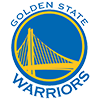Warriors
1.1% implied probability

+10000
100 to 1Pelicans
0.8% implied probability

+10000
100 to 1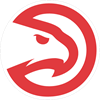Hawks
0.8% implied probability

+10000
100 to 1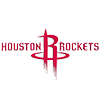Rockets
0.8% implied probability

+15000
150 to 1Grizzlies
0.5% implied probability

+15000
150 to 1Magic
0.5% implied probability

+15000
150 to 1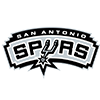Spurs
0.5% implied probability

+25000
250 to 1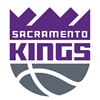Kings
0.3% implied probability

+25000
250 to 1Hornets
0.3% implied probability

+25000
250 to 1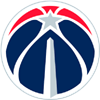Wizards
0.3% implied probability

+50000
500 to 1Timberwolves
0.2% implied probability

+50000
500 to 1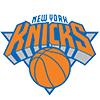Knicks
0.2% implied probability

+50000
500 to 1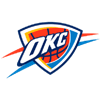Thunder
0.2% implied probability

+50000
500 to 1Pistons
0.2% implied probability

+50000
500 to 1Cavaliers
0.2% implied probability

+50000
500 to 1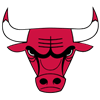Bulls
0.2% implied probability